• +91 9971497814
• info@interviewmaterial.com

# Chapter 8 Binomial Theorem Ex-8.1 Interview Questions Answers

### Related Subjects

Question 1 : Expand the expression (1– 2x)5

Answer 1 : By using Binomial Theorem, the expression (1– 2x)5 can be expanded as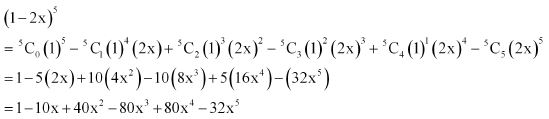Question 2 : Expand the expression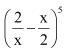By using Binomial Theorem, the expressioncan be expanded as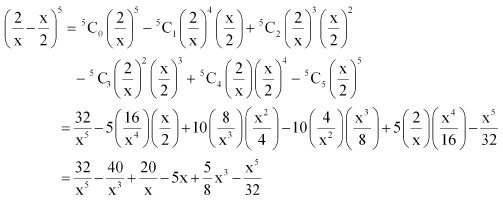Question 3 : Expand the expression (2x – 3)6

Answer 3 : By using Binomial Theorem, the expression (2x – 3)6 can be expanded as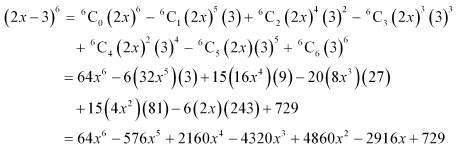Question 4 : Expand the expression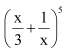By using Binomial Theorem, the expressioncan be expanded as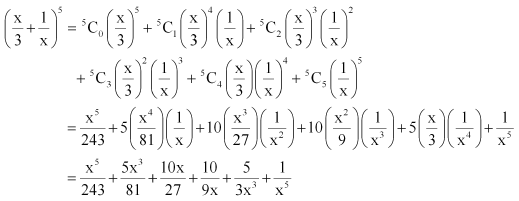Question 5 : Expand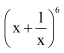Answer 5 : By using Binomial Theorem, the expressioncan be expanded as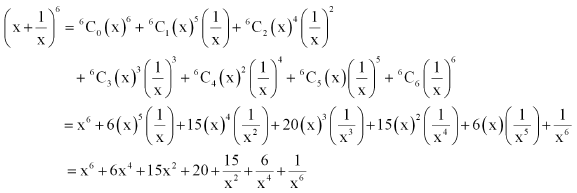Question 6 : Using Binomial Theorem, evaluate (96)3

96 can be expressed as the sum or difference of two numbers whose powers are easier to calculate and then, binomial theorem can be applied.
It can be written that, 96 = 100 – 4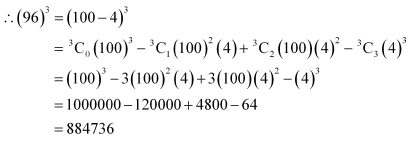Question 7 : Using Binomial Theorem, evaluate (102)5

102 can be expressed as the sum or difference of two numbers whose powers are easier to calculate and then, Binomial Theorem can be applied.
It can be written that, 102 = 100 + 2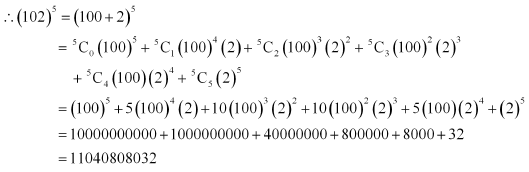Question 8 : Using Binomial Theorem, evaluate (101)4

101 can be expressed as the sum or difference of two numbers whose powers are easier to calculate and then, Binomial Theorem can be applied.
It can be written that, 101 = 100 + 1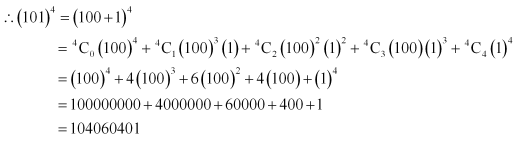Question 9 : Using Binomial Theorem, evaluate (99)5

99 can be written as the sum or difference of two numbers whose powers are easier to calculate and then, Binomial Theorem can be applied.
It can be written that, 99 = 100 – 1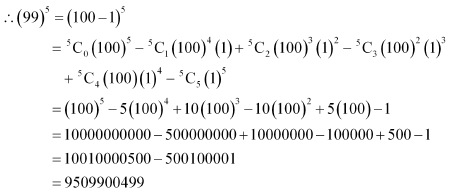Question 10 :

Using Binomial Theorem, indicate which number is larger (1.1)10000 or 1000.

By splitting 1.1 and then applying Binomial Theorem,the first few terms of (1.1)10000 canbe obtained as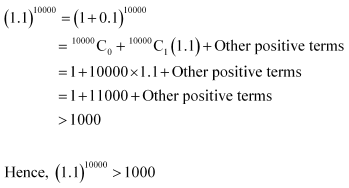Todays Deals### Chapter 8 Binomial Theorem Ex-8.1 Contributorskrishan

Name:
Email:

# Latest News# 9000 interview questions in different categories